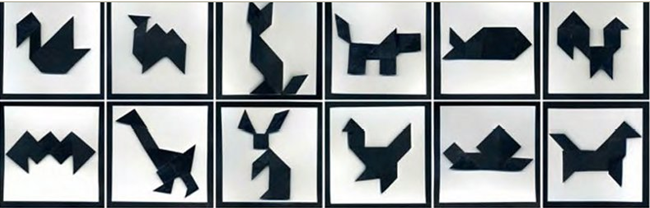Maths
Design
Tangrams
Author
Marion Montocchio, Lycée Charles Baudelaire
Target Group
12-17 years old students
Brief Description
Tangrams

Share

TANGRAM

DAY 1

Sheet « how to make a tangram”

Class :   Read and follow the instructions while the teacher builds a tangram

Write geometric vocabulary

Sheet « geometry”

(square, triangle, right (angle) triangle, iscosceles triangle, trapezoid, parallelogram

And complete the voc sheet)

Groups of 2 :     Read and follow the instructions to build a tangram

Try to make the big square again

Write a text to explain how to build the big square

Write vocabulary on black board :           on the top, at the bottom, on the left/ right

Above, below

On the left/right hand corner

Small medium big

On, along

Be carefull with the “tricky” parallelogram

DAY 2

Class :give instructions to teacher to build the big square

Write voc again on board

Groups of 2:  Give an outline to each group

Try to find the shape

Check with correction

Write a text to explain how to build the shape (without giving the name of the shape)

DAY 3

Exchange texts and try to follow the instructions (is it sufficient ? what is missing ?)

# How to Make a Tangram

## Things You'll Need:

• Paper

• scissors

• marker or pen

## Instructions

Begin with a square of paper.

Cut the square into two large isosceles right triangles.

Fold one of these triangles in half and cut it. Cut it right in the middle of it.

Fold the point of the other large triangle in order to get an isosceles right triangle and an isosceles trapezoid. Cut along the fold.

Fold the larger piece, the isosceles trapezoid, in half and cut it into two pieces : you get two right trapezoid.

Fold one of the right trapezoid, and cut it, in order to get a small square and a small isosceles right triangle.

Fold the other right trapezoid, and cut it, in order to get a small isosceles right triangle and a parallelogram.

Now, see if you can put the tans together to form a square.

Other tangram shapes :# Instructions

Start with the medium triangle. Put it at the bottom,  the hypotenuse down.

On the right of the medium triangle, put the parallelogram, its longer side, at the bottom.

Put the square on the other side, on the left. On right angle of the square on the right angle of the triangle. The two right angles are making a straight angle.

Along the side of this straight angle put a big right angle triangle, its hypotenuse touching the straight angle. The right angle of the right triangle is now on the up right corner.

Put one of the two big triangles on top, the hypotenuse up.

Put the second big triangles on left on the first one, the hypotenuse facing left, two sides of the big triangles are touching each other.

We built half of the square

Then put one of the two small triangle hypotenuse down, on the left bottom corner of the square.

Put the square, touching the small and big triangle on the top and right of the small triangle.

Put the second small triangle symmetrically of the other small triangle with the square. (hypotenuse facing right and vertically, touching the square and the big triangle.

Put the parallelogram on the right (the big side of the parallelogram touching the hypotenuse of the small triangle)

Put the medium triangle on the bottom right corner to complete the square.

TANGRAM MESSAGE

(color :________________________)

## Related files

 00 tangram description 299 KB 01 basic square 145 KB 02 tangram shapes 164 KB 03 instructions 160 KB 04 message 290 KB _organisation 325 KB
If you have tried out a lesson, please fill the next form to help us to improve it: